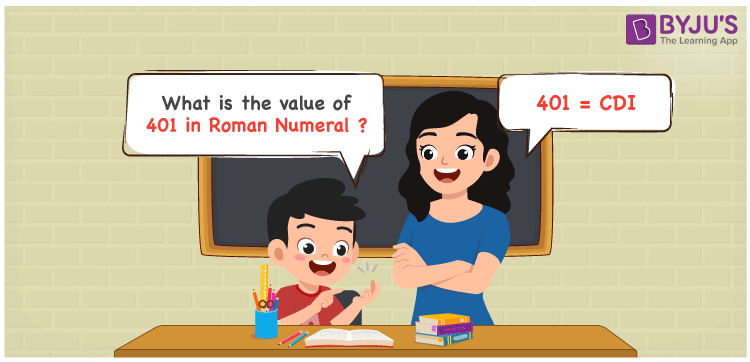# 401 in Roman Numerals

401 in Roman Numerals is CDI. Any Roman numeral uses only the 7 primary symbols which are I, V, X, L, C, D, and M. Here C represents 100 and D represents 500. The difference between 500 and 100, can give the number 400 and 1 is added to make the sum as 401. For understanding the rules on Roman Numerals, you can refer to the link Rules to write Roman Numerals. The number 401 is written as CDI. The details on how to write 401 is given in the next section of this article.

 Number Roman Numeral 401 CDI

## How to Write 401 in Roman Numerals?To convert 401 in Roman Numerals, we need to represent 401 as the difference of these fundamental symbols. The representation of 400 in terms of 100 will be against the rule of Roman numerals and hence difference is used.

It can be done as 500 – 100 ( 500 = D and 100 = C). Therefor 401 will be;

401 = 400 + 1

401 = (500 – 100) + 1

401 = (D – C) + I.

401 = CDI

CDI = 401.

## Video Lesson on Roman Numerals## Frequently Asked Questions on 401 in Roman Numerals

Q1

### How to write the number 401 in Roman Numerals?

401 in Roman Numerals is written as CDI.
Q2

### Do CDI and IDC represent Roman Numerals?

No. CDI represents Roman Numerals and it is the number 401, whereas IDC is an invalid Roman Numeral.
Q3

### How to express the number Four Hundred and One in Roman numerals?

The number Four Hundred and One is expressed as CDI in Roman Numerals.